Home
Hostname: page-component-768dbb666b-x9ds4 Total loading time: 0.752 Render date: 2023-02-06T20:30:30.550Z Has data issue: true Feature Flags: { "useRatesEcommerce": false } hasContentIssue trueForum of Mathematics, Sigma

# EXPONENTIAL IMPROVEMENT IN PRECISION FOR SIMULATING SPARSE HAMILTONIANS

Published online by Cambridge University Press:  02 March 2017

## Abstract

HTML view is not available for this content. However, as you have access to this content, a full PDF is available via the ‘Save PDF’ action button.

We provide a quantum algorithm for simulating the dynamics of sparse Hamiltonians with complexity sublogarithmic in the inverse error, an exponential improvement over previous methods. Specifically, we show that a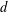$d$-sparse Hamiltonian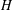$H$ acting on$n$ qubits can be simulated for time$t$ with precision$\unicode[STIX]{x1D716}$ using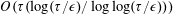$O(\unicode[STIX]{x1D70F}(\log (\unicode[STIX]{x1D70F}/\unicode[STIX]{x1D716})/\log \log (\unicode[STIX]{x1D70F}/\unicode[STIX]{x1D716})))$ queries and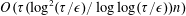$O(\unicode[STIX]{x1D70F}(\log ^{2}(\unicode[STIX]{x1D70F}/\unicode[STIX]{x1D716})/\log \log (\unicode[STIX]{x1D70F}/\unicode[STIX]{x1D716}))n)$ additional 2-qubit gates, where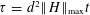$\unicode[STIX]{x1D70F}=d^{2}\Vert H\Vert _{\max }t$. Unlike previous approaches based on product formulas, the query complexity is independent of the number of qubits acted on, and for time-varying Hamiltonians, the gate complexity is logarithmic in the norm of the derivative of the Hamiltonian. Our algorithm is based on a significantly improved simulation of the continuous- and fractional-query models using discrete quantum queries, showing that the former models are not much more powerful than the discrete model even for very small error. We also simplify the analysis of this conversion, avoiding the need for a complex fault-correction procedure. Our simplification relies on a new form of ‘oblivious amplitude amplification’ that can be applied even though the reflection about the input state is unavailable. Finally, we prove new lower bounds showing that our algorithms are optimal as a function of the error.

## MSC classification

Type
Research Article
Creative CommonsThis is an Open Access article, distributed under the terms of the Creative Commons Attribution licence (http://creativecommons.org/licenses/by/4.0/), which permits unrestricted re-use, distribution, and reproduction in any medium, provided the original work is properly cited.

## References

Aharonov, D. and Ta-Shma, A., ‘Adiabatic quantum state generation and statistical zero knowledge’, inProceedings of the 35th ACM Symposium on Theory of Computing (ACM, New York, 2003), 2029.Google Scholar
Ambainis, A., Childs, A. M., Reichardt, B. W., Špalek, R. and Zhang, S., ‘Any AND-OR formula of size N can be evaluated in time N 1/2+o (1) on a quantum computer’, SIAM J. Comput. 39(6) (2010), 25132530.CrossRefGoogle Scholar
Ambainis, A., Magnin, L., Roetteler, M. and Roland, J., ‘Symmetry-assisted adversaries for quantum state generation’, inProceedings of the 26th IEEE Conference on Computational Complexity (IEEE, Piscataway, NJ, 2011), 167177.Google Scholar
Babbush, R., Berry, D. W., Kivlichan, I. D., Wei, A. Y., Love, P. J. and Aspuru-Guzik, A., ‘Exponentially more precise quantum simulation of fermions II: Quantum chemistry in the CI matrix representation’, Preprint, 2015, arXiv:1506.01029.Google Scholar
Babbush, R., Berry, D. W., Kivlichan, I. D., Wei, A. Y., Love, P. J. and Aspuru-Guzik, A., ‘Exponentially more precise quantum simulation of fermions in second quantization’, New J. Phys. 18 (2016), 033032.CrossRefGoogle Scholar
Beals, R., Buhrman, H., Cleve, R., Mosca, M. and de Wolf, R., ‘Quantum lower bounds by polynomials’, J. ACM 48(4) (2001), 778797.CrossRefGoogle Scholar
Berry, D. W., Ahokas, G., Cleve, R. and Sanders, B. C., ‘Efficient quantum algorithms for simulating sparse Hamiltonians’, Commun. Math. Phys. 270(2) (2007), 359371.CrossRefGoogle Scholar
Berry, D. W. and Childs, A. M., ‘Black-box Hamiltonian simulation and unitary implementation’, Quantum Inf. Comput. 12(1–2) (2012), 2962.Google Scholar
Berry, D. W., Childs, A. M., Cleve, R., Kothari, R. and Somma, R. D., ‘Exponential improvement in precision for simulating sparse Hamiltonians’, inProceedings of the 46th ACM Symposium on Theory of Computing (ACM, New York, 2014), 283292.Google Scholar
Berry, D. W., Childs, A. M., Cleve, R., Kothari, R. and Somma, R. D., ‘Simulating Hamiltonian dynamics with a truncated Taylor series’, Phys. Rev. Lett. 114(9) (2015), 090502.CrossRefGoogle ScholarPubMed
Berry, D. W., Childs, A. M. and Kothari, R., ‘Hamiltonian simulation with nearly optimal dependence on all parameters’, inProceedings of the 56th IEEE Symposium on Foundations of Computer Science (IEEE, Piscataway, NJ, 2015), 792809.Google Scholar
Berry, D. W., Cleve, R. and Gharibian, S., ‘Gate-efficient discrete simulations of continuous-time quantum query algorithms’, Quantum Inf. Comput. 14(1–2) (2014), 130.Google Scholar
Childs, A. M., ‘Quantum information processing in continuous time’, PhD Thesis, Massachusetts Institute of Technology, 2004.Google Scholar
Childs, A. M., ‘On the relationship between continuous- and discrete-time quantum walk’, Comm. Math. Phys. 294(2) (2010), 581603.CrossRefGoogle Scholar
Childs, A. M., Cleve, R., Deotto, E., Farhi, E., Gutmann, S. and Spielman, D. A., ‘Exponential algorithmic speedup by quantum walk’, inProceedings of the 35th ACM Symposium on Theory of Computing (ACM, New York, 2003), 5968.Google Scholar
Childs, A. M., Cleve, R., Jordan, S. P. and Yonge-Mallo, D., ‘Discrete-query quantum algorithm for NAND trees’, Theory Comput. 5(5) (2009), 119123.CrossRefGoogle Scholar
Childs, A. M., Gosset, D. and Webb, Z., ‘Universal computation by multi-particle quantum walk’, Science 339(6121) (2013), 791794.CrossRefGoogle Scholar
Childs, A. M. and Kothari, R., ‘Simulating sparse Hamiltonians with star decompositions’, inTheory of Quantum Computation, Communication, and Cryptography (TQC 2010), Lecture Notes in Computer Science, 6519 (Springer, Berlin, Heidelberg, 2011), 94103.CrossRefGoogle Scholar
Childs, A. M. and Wiebe, N., ‘Hamiltonian simulation using linear combinations of unitary operations’, Quantum Inf. Comput. 12(11–12) (2012), 901924.Google Scholar
Christandl, M., Datta, N., Ekert, A. and Landahl, A. J., ‘Perfect state transfer in quantum spin networks’, Phys. Rev. Lett. 92(18) (2004), 187902.CrossRefGoogle ScholarPubMed
Clader, B. D., Jacobs, B. C. and Sprouse, C. R., ‘Preconditioned quantum linear system algorithm’, Phys. Rev. Lett. 110(25) (2013), 250504.CrossRefGoogle ScholarPubMed
Cleve, R., Gottesman, D., Mosca, M., Somma, R. D. and Yonge-Mallo, D., ‘Efficient discrete-time simulations of continuous-time quantum query algorithms’, inProceedings of the 41st ACM Symposium on Theory of Computing (ACM, New York, 2009), 409416.Google Scholar
Farhi, E., Goldstone, J. and Gutmann, S., ‘A quantum algorithm for the Hamiltonian NAND tree’, Theory Comput. 4(8) (2008), 169190.CrossRefGoogle Scholar
Farhi, E., Goldstone, J., Gutmann, S. and Sipser, M., ‘Limit on the speed of quantum computation in determining parity’, Phys. Rev. Lett. 81(24) (1998), 54425444.CrossRefGoogle Scholar
Farhi, E. and Gutmann, S., ‘Analog analogue of a digital quantum computation’, Phys. Rev. A 57(4) (1998), 24032406.CrossRefGoogle Scholar
Feynman, R. P., ‘Simulating physics with computers’, Int. J. Theor. Phys. 21(6) (1982), 467488.CrossRefGoogle Scholar
Harrow, A. W., Hassidim, A. and Lloyd, S., ‘Quantum algorithm for linear systems of equations’, Phys. Rev. Lett. 103(15) (2009), 150502.CrossRefGoogle Scholar
Huyghebaert, J. and Raedt, H. D., ‘Product formula methods for time-dependent Schrödinger problems’, J. Phys. A 23(24) (1990), 5777.CrossRefGoogle Scholar
Jordan, C., ‘Essai sur la géométrie à n dimensions’, Bull. Soc. Math. France 3 (1875), 103174.CrossRefGoogle Scholar
Lee, T., Mittal, R., Reichardt, B. W., Špalek, R. and Szegedy, M., ‘Quantum query complexity of state conversion’, inProceedings of the 52nd IEEE Symposium on Foundations of Computer Science (IEEE, Piscataway, NJ, 2011), 344353.Google Scholar
Lloyd, S., ‘Universal quantum simulators’, Science 273(5278) (1996), 10731078.CrossRefGoogle ScholarPubMed
Marriott, C. and Watrous, J., ‘Quantum Arthur–Merlin games’, Comput. Complexity 14(2) (2005), 122152.CrossRefGoogle Scholar
Mochon, C., ‘Hamiltonian oracles’, Phys. Rev. A 75(4) (2007), 042313.CrossRefGoogle Scholar
Motwani, R. and Raghavan, P., Randomized Algorithms (Cambridge University Press, 1995).CrossRefGoogle Scholar
Nagaj, D., Wocjan, P. and Zhang, Y., ‘Fast amplification of QMA’, Quantum Inf. Comput. 9(11–12) (2009), 10531068.Google Scholar
Paetznick, A. and Svore, K. M., ‘Repeat-until-success: Non-deterministic decomposition of single-qubit unitaries’, Quantum Inf. Comput. 14(15–16) (2014), 12771301.Google Scholar
Poulin, D., Qarry, A., Somma, R. D. and Verstraete, F., ‘Quantum simulation of time-dependent Hamiltonians and the convenient illusion of Hilbert space’, Phys. Rev. Lett. 106(17) (2011), 170501.CrossRefGoogle ScholarPubMed
Scherer, A., Valiron, B., Mau, S.-C., Alexander, S., van den Berg, E. and Chapuran, T. E., ‘Concrete resource analysis of the quantum linear system algorithm used to compute the electromagnetic scattering cross section of a 2D target’, Quantum Inf. Process. 16(3) (2017), 60.CrossRefGoogle Scholar
Suzuki, M., ‘General theory of fractal path integrals with applications to many-body theories and statistical physics’, J. Math. Phys. 32(2) (1991), 400407.CrossRefGoogle Scholar
Watrous, J., ‘Zero-knowledge against quantum attacks’, SIAM J. Comput. 39(1) (2009), 2538.CrossRefGoogle Scholar
Watson, G. N., A Treatise on the Theory of Bessel Functions (Cambridge University Press, Cambridge, UK, 1922).Google Scholar
Wiebe, N., Berry, D. W., Høyer, P. and Sanders, B. C., ‘Simulating quantum dynamics on a quantum computer’, J. Phys. A 44(44) (2011), 445308.CrossRefGoogle Scholar
Wiebe, N. and Roetteler, M., ‘Quantum arithmetic and numerical analysis using Repeat-Until-Success circuits’, Quantum Inf. Comput. 16(1–2) (2016), 134178.Google ScholarYou have AccessOpen access
10
Cited by

# Save article to Kindle

Note you can select to save to either the @free.kindle.com or @kindle.com variations. ‘@free.kindle.com’ emails are free but can only be saved to your device when it is connected to wi-fi. ‘@kindle.com’ emails can be delivered even when you are not connected to wi-fi, but note that service fees apply.

Find out more about the Kindle Personal Document Service.

EXPONENTIAL IMPROVEMENT IN PRECISION FOR SIMULATING SPARSE HAMILTONIANS
Available formats
×

# Save article to Dropbox

To save this article to your Dropbox account, please select one or more formats and confirm that you agree to abide by our usage policies. If this is the first time you used this feature, you will be asked to authorise Cambridge Core to connect with your Dropbox account. Find out more about saving content to Dropbox.

EXPONENTIAL IMPROVEMENT IN PRECISION FOR SIMULATING SPARSE HAMILTONIANS
Available formats
×

# Save article to Google Drive

To save this article to your Google Drive account, please select one or more formats and confirm that you agree to abide by our usage policies. If this is the first time you used this feature, you will be asked to authorise Cambridge Core to connect with your Google Drive account. Find out more about saving content to Google Drive.

EXPONENTIAL IMPROVEMENT IN PRECISION FOR SIMULATING SPARSE HAMILTONIANS
Available formats
×
×